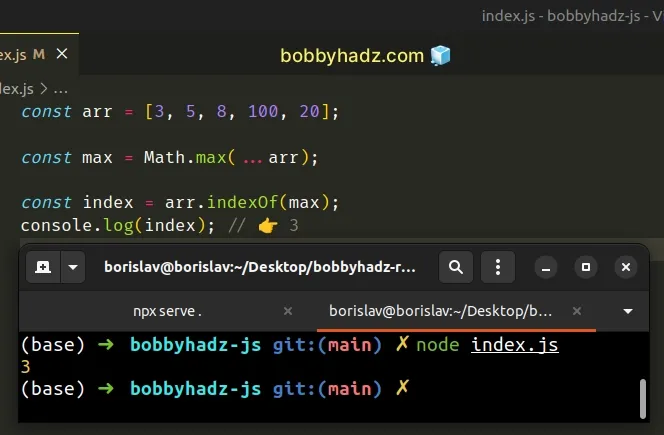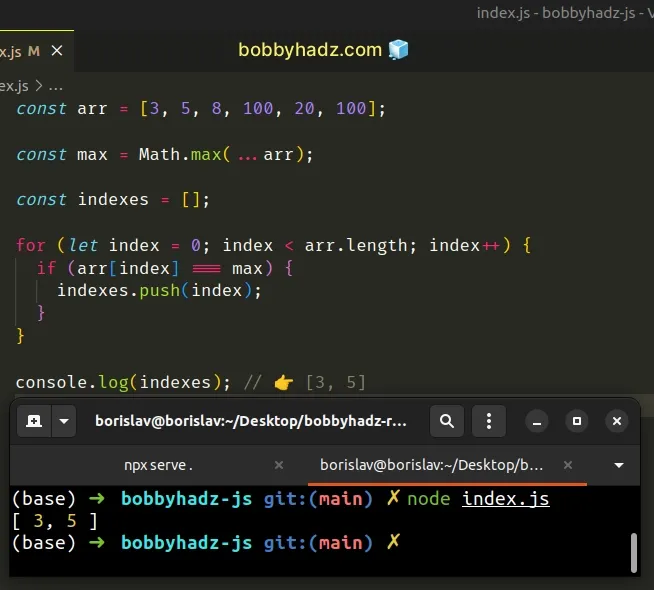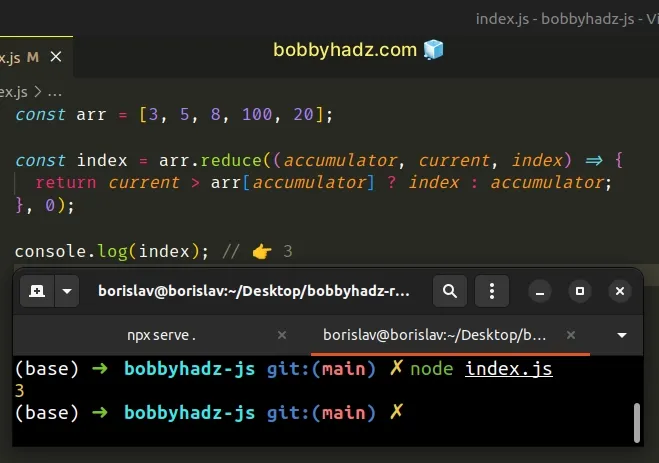# Get the Index of the Max/Min value in Array in JavaScriptLast updated: Dec 21, 2022
5 min## #Get the Index of the Max value in an Array in JavaScript

To get the index of the max value in an array:

1. Use the `Math.max()` method to get the max value in the array.
2. Use the `String.indexOf()` method to get the index of the max value.
3. The `indexOf()` method returns the index of the value in the array or `-1` if the value isn't found.
index.js
```Copied!```const arr = [3, 5, 8, 100, 20];

const max = Math.max(...arr);

const index = arr.indexOf(max);
console.log(index); // 👉️ 3
``````If you need to get the index of the min value, use the `Math.min()` function instead.

index.js
```Copied!```const arr = [10, 5, 0, 15, 30];

const min = Math.min(...arr);

const index = arr.indexOf(min);
console.log(index); // 👉️ 2
``````

We used the Math.max() method to get the max value in the array.

The `Math.max()` method expects multiple, comma-separated numbers as arguments, so we can't just pass it an array directly.

index.js
```Copied!```const max = Math.max(3, 5, 8)
console.log(max) // 👉️ 8
``````

We used the spread operator (...) to unpack the values of the array and passed them as multiple, comma-separated arguments to the `Math.max()` method.

index.js
```Copied!```const arr = [3, 5, 8, 100, 20];

const max = Math.max(...arr);

const index = arr.indexOf(max);
console.log(index); // 👉️ 3
``````

The last step is to use the Array.indexOf method to get the index of the `max` value.

If we had multiple array elements with the same max value, the `indexOf` method would return only the index of the first occurrence.

You can use a `for` loop to get the index of all max values in the array.

## #Get the indexes of all elements with the max value in an array

To get the indexes of all elements with the max value in an array:

1. Use the `Math.max()` method to get the max value in the array.
2. Declare an `indexes` variable that stores an empty array.
3. Iterate over the array and push only the indexes of the max values to the `indexes` array.
index.js
```Copied!```const arr = [3, 5, 8, 100, 20, 100];

const max = Math.max(...arr);

const indexes = [];

for (let index = 0; index < arr.length; index++) {
if (arr[index] === max) {
indexes.push(index);
}
}

console.log(indexes) // 👉️ [3, 5]
``````We used a for loop to iterate for `array.length` iterations.

On each iteration, we check if the element at that index is the max value, and if it is, we push the current index to the `indexes` array.

The `indexes` array stores the index of all occurrences of the `max` value in the array.

You can also use the `Array.reduce()` method.

## #Get the Index of the Max value in an Array using Array.reduce()

This is a three-step process:

1. Use the `Array.reduce()` method to iterate over the array.
2. Check if the current element is greater than the accumulator.
3. If the condition is met, return the current index, otherwise, return the accumulator.
index.js
```Copied!```const arr = [3, 5, 8, 100, 20];

const index = arr.reduce((accumulator, current, index) => {
return current > arr[accumulator] ? index : accumulator;
}, 0);

console.log(index); // 👉️ 3
``````The function we passed to the `Array.reduce()` method gets called with the accumulator value, the current array element and the current index.

The second argument we passed to `Array.reduce()` is used as the initial value for the `accumulator` variable.

We used `0` as the initial value.

Whatever we return from the function gets set as the new value of the accumulator variable.

On each iteration, we check if the current list item is greater than the element at the `accumulator` index.

If the condition is met, the current index is returned, otherwise, the `accumulator` index is returned.

After the last iteration, the `index` variable stores the index of the max value in the array.

## #Get the Index of the Min value in an Array in JavaScript

To get the index of the min value in an array:

1. Use the `Math.min()` method to get the min value in the array.
2. Use the `Array.indexOf()` method to get the index of the min value.
3. The `indexOf` method returns the index of the first occurrence of the value in the array.
index.js
```Copied!```const arr = [10, 5, 0, 15, 30];

const min = Math.min(...arr);

const index = arr.indexOf(min);
console.log(index); // 👉️ 2
``````

We used the spread (...) operator when we called the Math.min() method.

The `Math.min()` method expects comma-separated numbers as arguments, so we can't directly pass it an array.
index.js
```Copied!```const min = Math.min(10, 5, 0)
console.log(min) // 👉️ 0
``````

We used the spread operator (...) to unpack the values of the array and passed them as multiple, comma-separated arguments to the `Math.min()` method.

We then used the Array.indexOf method to find the index of the first occurrence of the `min` value.

index.js
```Copied!```const arr = [10, 5, 0, 15, 30];

const min = Math.min(...arr);

const index = arr.indexOf(min);
console.log(index); // 👉️ 2
``````
If there were multiple array elements with the value of `0`, the `indexOf` method would return the index of the first occurrence.

If you need to find the index of all occurrences of the `min` value in the array, use a `for` loop.

index.js
```Copied!```const arr = [10, 5, 0, 15, 30, 0, 0];

const min = Math.min(...arr);
console.log(min); // 👉️ 0

const indexes = [];

for (let i = 0; i < arr.length; i++) {
if (arr[i] === min) {
indexes.push(i);
}
}

console.log(indexes); // 👉️ [2, 5, 6]
``````

We used the `Mah.min()` method to find the `min` value in the array and used a `for` loop to iterate over the array.

On each iteration, we check if the current array element is equal to the `min` value.

If the condition is met, we push the current index into the `indexes` array.

The `indexes` array stores the index of all occurrences of the `min` value in the array.

You can also use the `Function.apply` method as an alternative to the spread (...) operator.

## #Get the Index of the Min value in an Array using Function.apply()

This is a three-step process:

1. Use the `Function.apply()` method in the call to the `Math.min()` method.
2. Use the `Array.indexOf()` method to get the index of the min value.
3. The `indexOf` method returns the index of the first occurrence of the value in the array.
index.js
```Copied!```const arr = [10, 5, 0, 15, 30];

// 👇️ now using apply, instead of ...
const min = Math.min.apply(null, arr);

const index = arr.indexOf(min);
console.log(index); // 👉️ 2
``````

The only difference in the code is how we get the min value in the array.

The arguments we passed to the Function.apply() method are:

1. The `this` argument - for our purposes it is irrelevant.
2. The array whose values will be passed to the `Math.min()` method as multiple, comma-separated arguments.

Under the hood, the `apply` method unpacks the values of the array and passes them as multiple arguments to the function the method is called on.